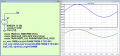# What does digital multimeter result show when measuring AC V ?

#### Günther_Guss

Joined Sep 23, 2023
1
Hello anyone, i'd like to ask something, What does digital multimeter result show when measuring AC V ? is it V ave or V rms ? Also what does it result show when the voltage source still has voltage ripples in it/or after being filtered by capacitors ? Is it V ave or V rms of the voltage ripple ? Whats the formula for this. Thanks.

#### Papabravo

Joined Feb 24, 2006
20,597
The average voltage of an AC signal will usually be zero. The most likely measurement will be RMS (Root Mean Square). The DMM will square the incoming waveform, take the average or mean value over one cycle, then take the square root of that mean squared value and display it. The algorithm is the same for any arbitrary waveform. So, a rectified and filtered AC waveform would produce an RMS value that includes both the DC and the AC components of the waveform.Last edited:

Joined Jul 18, 2013
28,024
VRMS when measuring an AC source.
Is the question regarding caps after rectification?
If not what/how is meant by 'filtered' ?

#### MrChips

Joined Oct 2, 2009
29,809
Welcome to AAC!

That is a very good question.
First of all, it depends on the meter. All meters are not the same, even ones that state TRUE RMS on the front face.

First some background is required.
Testmeters with a AC V range are meant to measure line voltages such as 100-120VAC and 200-240VAC at 50/60Hz.
AC V is always measured in RMS = root mean square. In general, all AC meters are frequency dependent, i.e. it depends on what frequency is being measured.

If you are measuring AC ripple superimposed on a DC voltage, almost all testmeters are incapable of measuring this. You need to look at this with an oscilloscope.

Now for some formulae.
When we think of AC, we need to first consider the basic sine wave formula.

v = DC + A x sin(2πft)
where DC = DC offset
A = signal amplitude = ½ peak-to-peak voltage
For this discussion, we assume DC = 0

RMS = √2 x A= 0.707 x A
This true only for a sinusoidal wave. This is why it is difficult for many testmeters to show RMS for anything that is not a sine wave.

The average voltage of a sine wave = 0
The average voltage of one-half of the cycle (assuming positive cycle) = 2 x A/π = 0.637 x A

If the signal is not sinusoidal you have to use different forumlae.

If you want to measure AC ripple from a power supply, you need to use an oscilloscope.
v = DC + A x sin(2πft)

You can remove the DC component by setting the oscilloscope channel input to AC.

•drjohsmith

#### Papabravo

Joined Feb 24, 2006
20,597
Welcome to AAC!

That is a very good question.
First of all, it depends on the meter. All meters are not the same, even ones that state TRUE RMS on the front face.

First some background is required.
Testmeters with a AC V range are meant to measure line voltages such as 100-120VAC and 200-240VAC at 50/60Hz.
AC V is always measured in RMS = root mean square. In general, all AC meters are frequency dependent, i.e. it depends on what frequency is being measured.

If you are measuring AC ripple superimposed on a DC voltage, almost all testmeters are incapable of measuring this. You need to look at this with an oscilloscope.

Now for some formulae.
When we think of AC, we need to first consider the basic sine wave formula.

v = DC + A x sin(2πft)
where DC = DC offset
A = signal amplitude = ½ peak-to-peak voltage
For this discussion, we assume DC = 0

RMS = √2 x A= 0.707 x A
This true only for a sinusoidal wave. This is why it is difficult for many testmeters to show RMS for anything that is not a sine wave.

The average voltage of a sine wave = 0
The average voltage of one have of the cycle (assuming positive cycle) = 2 x A/π = 0.637 x A

If the signal is not sinusoidal you have to use different forumlae.

If you want to measure AC ripple from a power supply, you need to use an oscilloscope.
v = DC + A x sin(2πft)

You can remove the DC component by setting the oscilloscope channel input to AC.
The basic RMS algorithm works even if the input is not a sinewave. It is a mathematical construct. For a sinewave source it produces the same result in computing power in a resistive load that a DC source of the same voltage as the RMS sinewave source would produce. This is not generally true for other waveform types, rending an RMS measurement of a non-sinewave source of dubious value.

#### crutschow

Joined Mar 14, 2008
33,331
As noted, there are two ways a multimeter measures AC voltage.

The ones that do expressly say "True RMS" generally measure the average value of the rectified AC waveform (0.637 of a sinewave peak voltage), then multiply that by a factor of 0.707/0.636 to display the RMS value.
Of course that's only exactly true for a pure sinewave.

The True RMS meters measure and display the true RMS value of the waveform, even if it is not a sinewave.

#### MrChips

Joined Oct 2, 2009
29,809
There is a computational method of calculating the RMS value that applies to any shape of waveform.

You take the square of the voltage, find the mean using integration, then take the square root.
That is why it is called root mean square.

Old fashioned hot wire ammeters display true RMS because they work on the heating effect of the current.

•JohnInTX and Papabravo